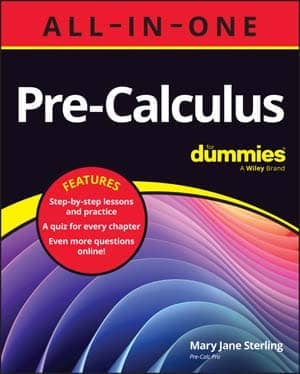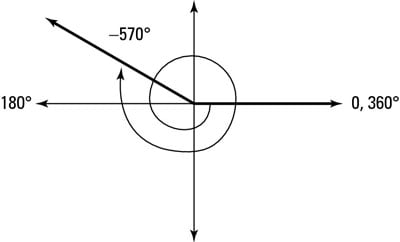##### Pre-Calculus All-in-One For DummiesMany times in your journey through trigonometry — actually, all the time — drawing a figure will help you solve a given problem. So what do you do if you're asked to draw an angle that has a measure greater than 360 degrees? Or a negative measure? How about both? Your head is probably spinning! No worries; the following steps will help.

Suppose that you need to draw a –570-degree angle. Here's what you do:

1. Find a co-terminal angle by adding 360 degrees.

Adding 360 degrees to –570 degrees gives you –210 degrees.

2. If the angle is still negative, keep adding 360 degrees until you get a positive angle in standard position.

Adding 360 degrees to –210 degrees gives you 150 degrees. This angle is 30 degrees less than 180 degrees (much closer to the 180 degrees line than 90 degrees).

3. Draw the angle you create in Step 2.A –570 degree angle on the coordinate plane.

You need to draw a –570 degrees angle, so be careful which way your arrow points and how many times you travel around the unit circle before you stop at the terminal side.

This angle starts at 0 on the x-axis and moves in clockwise because you're finding a negative angle. The figure shows what the finished angle looks like.